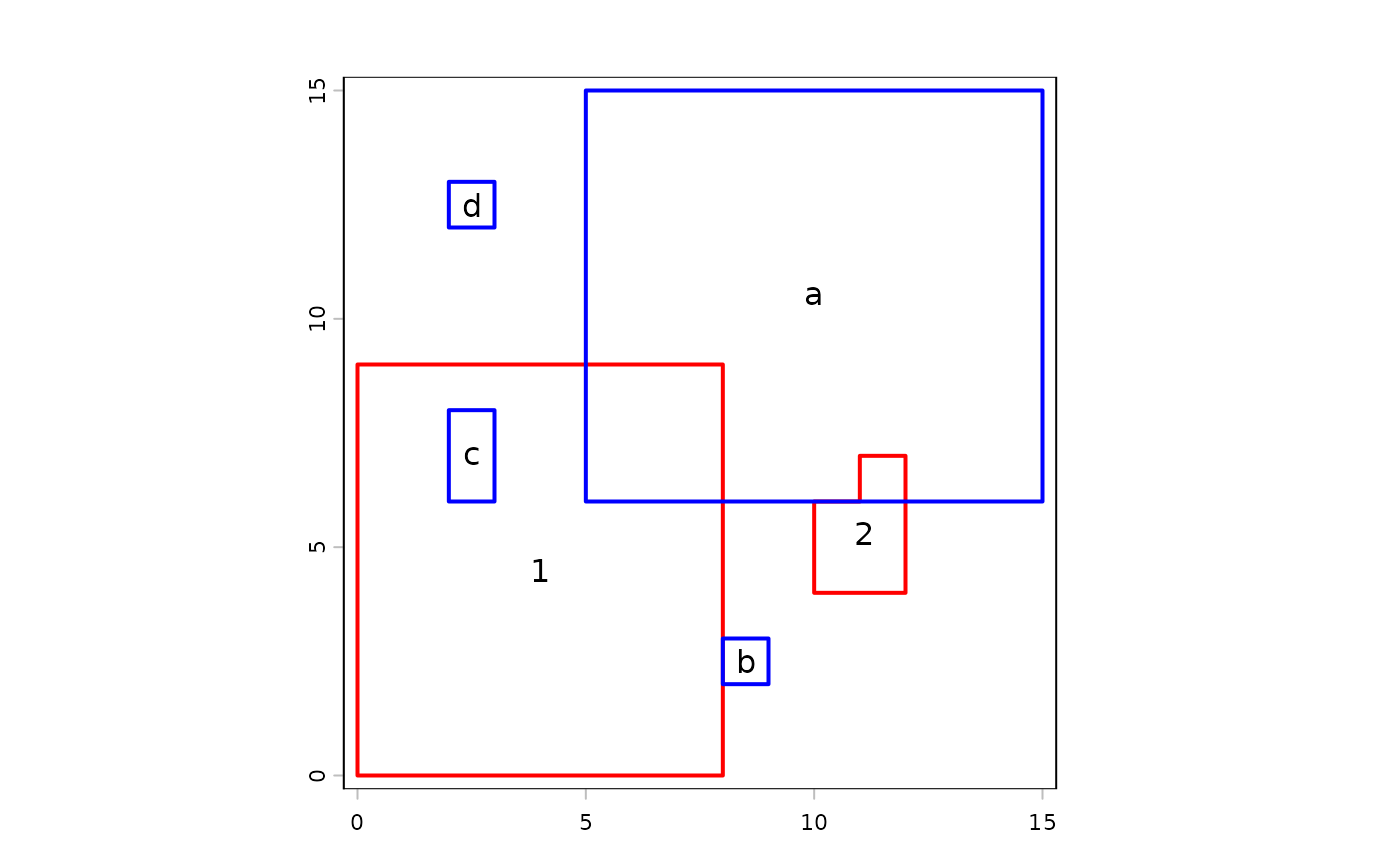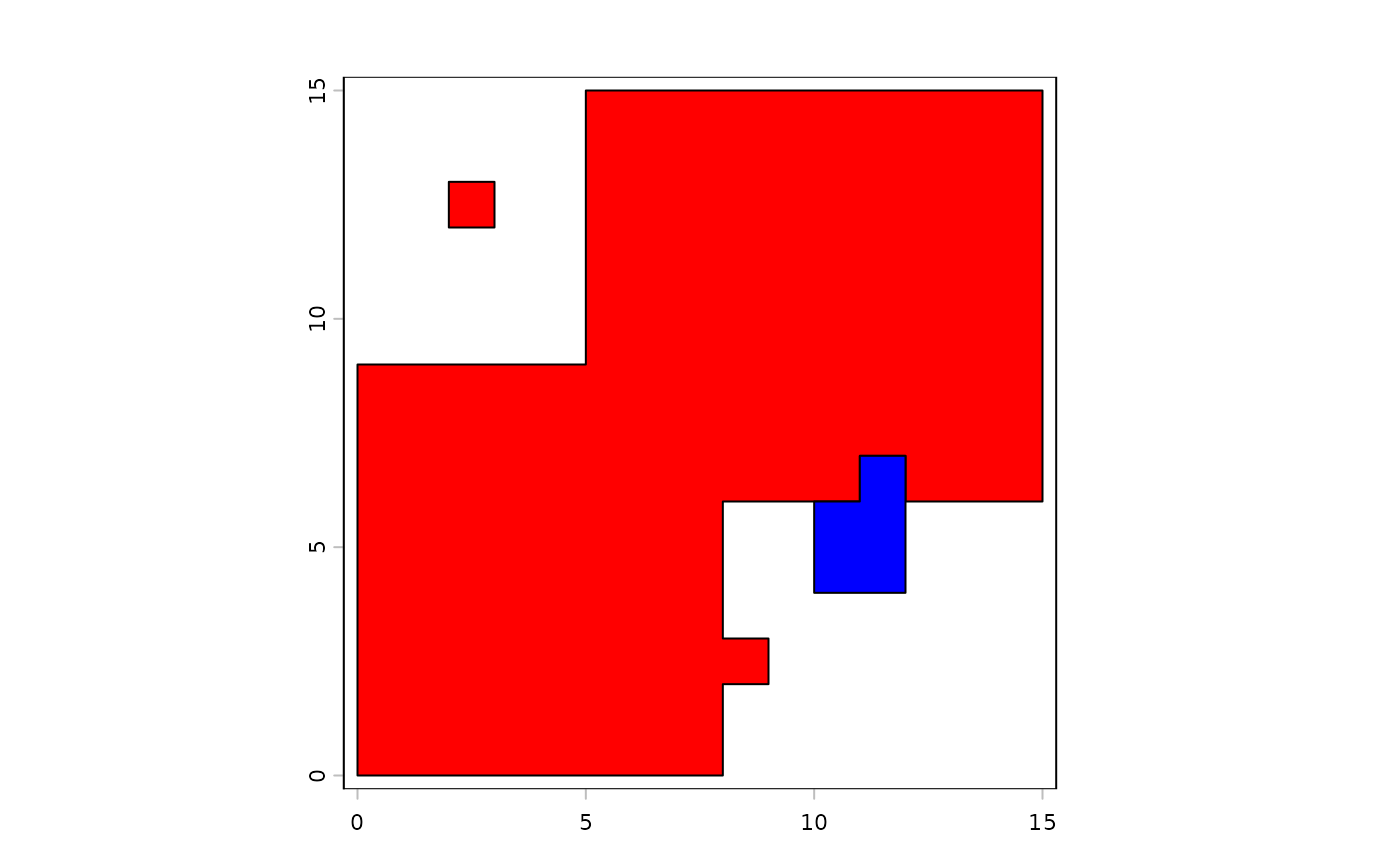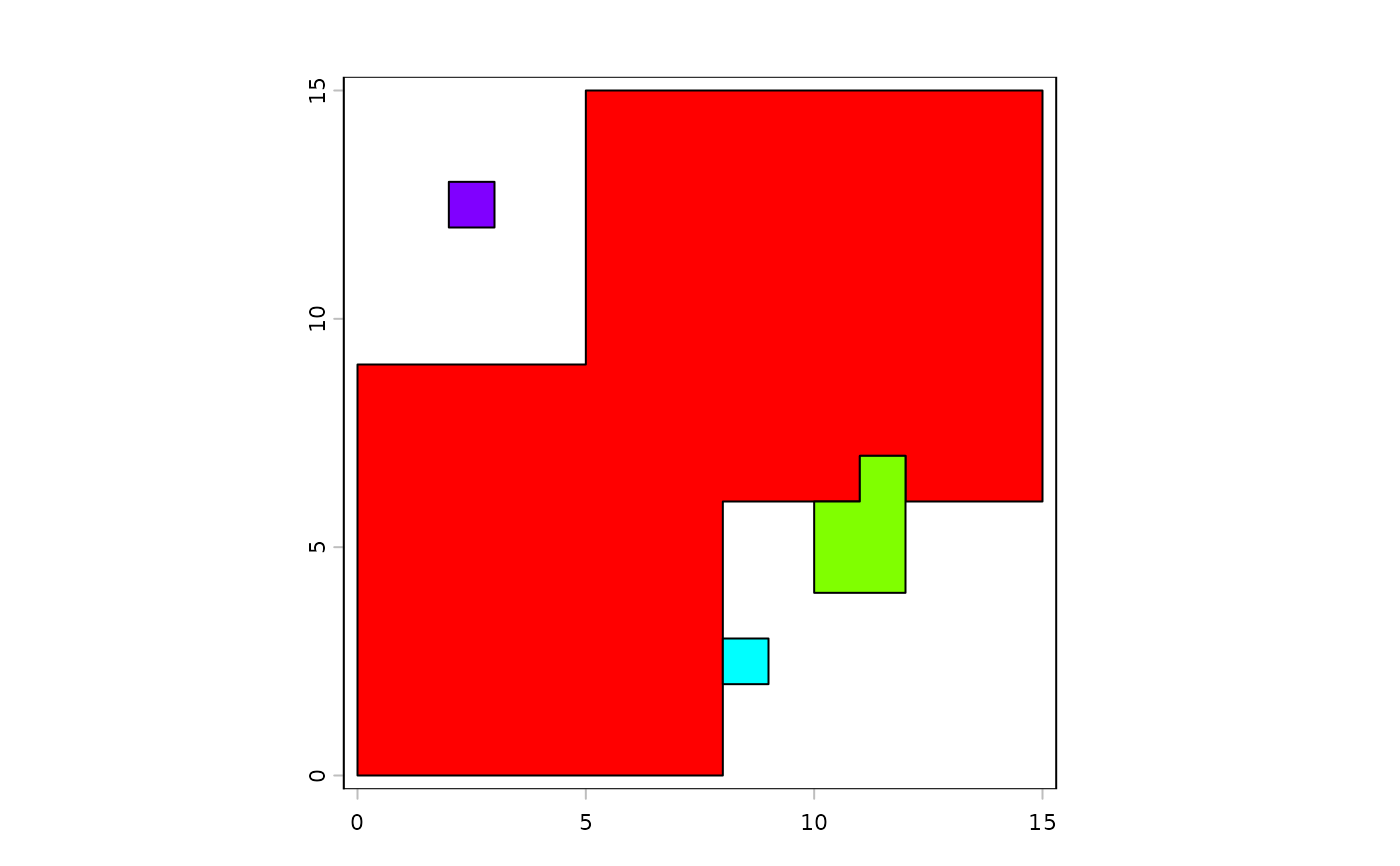Combine the geometries of one SpatVector with those of another. Geometries can be combined based on overlap, shared boundaries and distance (in that order of operation).

The typical use-case of this method is when you are editing geometries and you have a number of small polygons in one SpatVector that should be part of the geometries of the another SpatVector; perhaps because they were small holes inbetween the borders of two SpatVectors.

To append SpatVectors use rbind and see methods like intersect and union for "normal" polygons combinations.

# S4 method for SpatVector,SpatVector
combineGeoms(x, y, overlap=TRUE, boundary=TRUE, distance=TRUE,
append=TRUE, minover=0.1, maxdist=Inf, dissolve=TRUE, erase=TRUE)

## Arguments

x

SpatVector of polygons

y

SpatVector of polygons geometries that are to be combined with x

overlap

logical. If TRUE, a geometry is combined with the geometry it has most overlap with, if the overlap is above minover

boundary

logical. If TRUE, a geometry is combined with the geometry it has most shared border with

distance

logical. If TRUE, a geometry is combined with the geometry it is nearest to

append

logical. Should remaining geometries be appended to the output? Not relevant if distance=TRUE

minover

numeric

The fraction of the geometry in codey that overlaps with a geometry in x. Below this threshold, geometries are not considered overlapping

maxdist

numeric. Geometries further away from each other than this distance (in meters) will not be combined

dissolve

logical. Should internal boundaries be dissolved?

erase

logical. If TRUE no new overlapping areas are created

## Value

SpatVector

union, erase, intersect

sharedPaths, erase, intersect

## Examples

x1 <- vect("POLYGON ((0 0, 8 0, 8 9, 0 9, 0 0))")
x2 <- vect("POLYGON ((10 4, 12 4, 12 7, 11 7, 11 6, 10 6, 10 4))")

y1 <- vect("POLYGON ((5 6, 15 6, 15 15, 5 15, 5 6))")
y2 <- vect("POLYGON ((8 2, 9 2, 9 3, 8 3, 8 2))")
y3 <- vect("POLYGON ((2 6, 3 6, 3 8, 2 8, 2 6))")
y4 <- vect("POLYGON ((2 12, 3 12, 3 13, 2 13, 2 12))")

x <- rbind(x1, x2)
values(x) <- data.frame(xid=1:2)
crs(x) <- "+proj=utm +zone=1"

y <- rbind(y1, y2, y3, y4)
values(y) <- data.frame(yid=letters[1:4])
crs(y) <- "+proj=utm +zone=1"

plot(rbind(x, y), border=c(rep("red",2), rep("blue", 4)), lwd=2)
text(x, "xid")
text(y, "yid")v <- combineGeoms(x, y)
plot(v, col=c("red", "blue"))v <- combineGeoms(x, y, boundary=FALSE, maxdist=1, minover=.05)
plot(v, col=rainbow(4))